### 贪婪搜索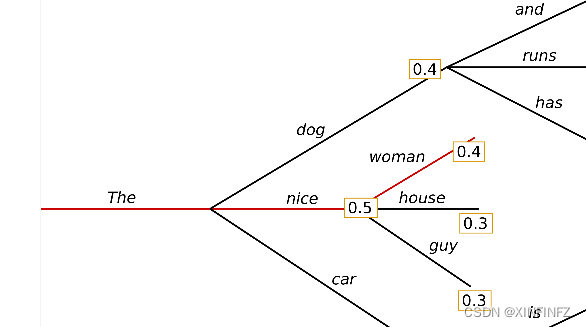``````from transformers import AutoModelForCausalLM, AutoTokenizer
import time
a1 = time.time()
checkpoint = "bigscience/bloom-1b1"

tokenizer = AutoTokenizer.from_pretrained(checkpoint)
model = AutoModelForCausalLM.from_pretrained(checkpoint)

inputs = tokenizer.encode("我和我的猫都很想你", return_tensors="pt") #prompt
outputs = model.generate(inputs,min_length=150,max_length=200)
print(tokenizer.decode(outputs)) #使用tokenizer对生成结果进行解码
a2 = time.time()
print(f'time cost is {a2 - a1} s')
``````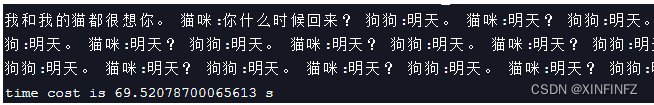### 粒子束搜索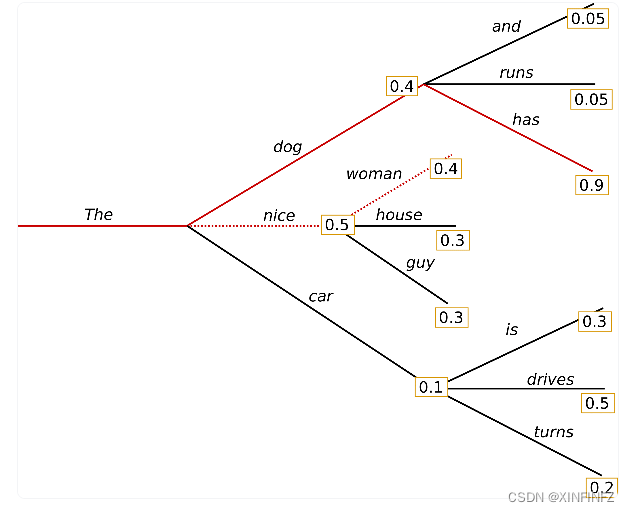``````outputs = model.generate(inputs,min_length=150,max_length=200,
num_beams=5,early_stopping=True)
``````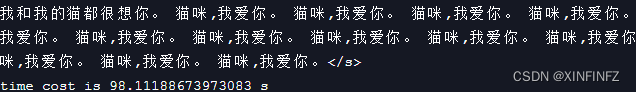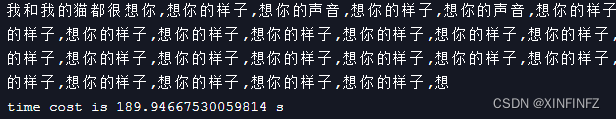### 限制粒子束搜索

``````outputs = model.generate(inputs,min_length=150,max_length=200,num_beams=5,
no_repeat_ngram_size=2,early_stopping=True)
``````

``````我和我的猫都很想你,想你的时候,我就会想起你。

time cost is 97.57852363586426 s
``````

``````from transformers import AutoModelForCausalLM, AutoTokenizer
import time
a1 = time.time()
checkpoint = "bigscience/bloom-1b1"
force_words = ["回家"] #强制词汇

tokenizer = AutoTokenizer.from_pretrained(checkpoint)
model = AutoModelForCausalLM.from_pretrained(checkpoint)
inputs = tokenizer.encode("我和我的猫都很想你", return_tensors="pt")
outputs = model.generate(inputs,force_words_ids=force_words_ids,min_length=150,max_length=200,num_beams=5,no_repeat_ngram_size=2,early_stopping=True)
print(tokenizer.decode(outputs))
a2 = time.time()
print(f'time cost is {a2 - a1} s')
``````

``````我和我的猫都很想你。 猫咪:你什么时候回来？

time cost is 115.72432279586792 s
``````

### 引用

https://huggingface.co/blog/how-to-generate
https://huggingface.co/docs/transformers/generation_strategies
https://huggingface.co/blog/constrained-beam-search